Wang, Y., Sun, Y., and Wu, K. (2023). “Investigation of the mechanical properties in the production process of biomass fuel pellets,” BioResources 18(2), 3560-3575.

#### Abstract

The compacting force in the biomass pelletizing process has remarkable effects on energy consumption, equipment life, and pellet quality. This paper presents an experimental study on the mechanical behavior for the pelletizing process of rice straw, wheat straw, and wood shavings, under different levels of technological parameters, including moisture content, compacting velocity, and particle size. Effects of these parameters on the constant coefficients in the three equations were analyzed. The relationship between the coefficients and the pelletizing process was considered. Results showed that Peiyun Huang equation was more suitable for the whole compacting process compared with the other two equations, which meant it was feasible to estimate the required input of the pelletizing system by measuring the product density based on the Peiyun Huang equation. No specific relationships between the coefficients and pellet quality and energy consumption were observed. It is infeasible to predict the pellet quality and energy consumption only by the mechanical properties of the biomass in densification process.

Investigation of the Mechanical Properties in the Production Process of Biomass Fuel Pellets

Yu Wang,* Yu Sun, and Kai Wu

The compacting force in the biomass pelletizing process has remarkable effects on energy consumption, equipment life, and pellet quality. This paper presents an experimental study on the mechanical behavior for the pelletizing process of rice straw, wheat straw, and wood shavings, under different levels of technological parameters, including moisture content, compacting velocity, and particle size. Effects of these parameters on the constant coefficients in the three equations were analyzed. The relationship between the coefficients and the pelletizing process was considered. Results showed that Peiyun Huang equation was more suitable for the whole compacting process compared with the other two equations, which meant it was feasible to estimate the required input of the pelletizing system by measuring the product density based on the Peiyun Huang equation. No specific relationships between the coefficients and pellet quality and energy consumption were observed. It is infeasible to predict the pellet quality and energy consumption only by the mechanical properties of the biomass in densification process.

DOI: 10.15376/biores.18.2.3560-3575

Keywords: Biomass; Densification; Pellets; Single pellet unit; Peiyun Huang equation

Contact information: Nanjing University of Science and Technology, School of Mechanical Engineering, 200 Xiaolingwei, Nanjing, Jiangsu 210094, People’s Republic of China;

*Corresponding author: wy@njust.edu.cn

INTRODUCTION

As a renewable resource, biomass pellets play an important role in energy security and in efforts to reduce greenhouse gas (GHG) emissions (Sikkema et al. 2021). The pellets are made from forest or agricultural residues by a pelletizing process, and they are easily handled because of their regular shape and higher density compared with the raw materials (Cui et al. 2021). Major technological challenges in the biomass pelletizing process are to maintain a stable pelletizing process with low energy consumption and production of high value-added pellets (Holm et al. 2006). Plenty of studies have been conducted on how to reduce energy consumption and improve pellet quality.

Most studies have focused on the influence of technological parameters on the pelletizing process. It was found that the parameters, such as temperature (Cui et al. 2021; Wilczyński et al. 2021), moisture content (Zhang et al. 2019; Lavergne et al. 2021), particle size (Guo et al. 2016; Chen et al. 2021), die dimensions (Xia et al. 2016; Dao et al. 2022), and compacting velocity (Wang et al. 2020a), etc., had noticeable effects on the pellet density, mechanical durability, and energy consumption. The optimal ranges of these parameters varied from each other for different materials. Therefore, similar experiments and optimization processes had to be conducted for new biomass materials. However, the material types changed frequently due to an increasing attention on the pretreatment of raw materials such as torrefaction (Sarker et al. 2022) and additive addition (Akbar et al. 2021; García et al. 2021). Thus, researchers have begun to look for a more widely applicable model for the biomass pelletizing process. A potential solution is to investigate the mechanical properties in the pelletizing process.

Holm et al. (2006, 2007) analyzed the compacting force in a single pellet channel based on an elastic theory and verified it with a single pellet unit. Results showed that the compacting force was affected by the friction coefficient, size of the pellet channel, and pre-stress pressure. Mani et al. (2004) applied three compaction equations to the densification process of four biomass materials. Results showed that the Heckel equation did not fit the data well. The dominant compaction mechanisms were rearrangement of particles, elastic deformation, and plastic deformation. Kaliyan and Morey (2009) developed a constitutive model to predict the compression behaviors of corn stover and switchgrass grinds; the pellet strength and durability were affected by the elastic modulus and viscous coefficient. Chevanan et al. (2010) investigated the compaction behavior of switchgrass, wheat straw, and corn stover. Results showed that the compaction behavior had remarkable effects on the bulk density. Granado et al. (2021) observed that the density and resistance of cassava waste briquettes increased with the compacting pressure. Meng et al. (2021) investigated the effects of ultrasonic vibration on biochar pelletizing process. They found that the periodic compacting force changed the chemical structures, compositions, and surface structure of biochar pellets, and had remarkable effects on the yield. Caicedo-Zuñiga et al. (2021) developed a visco-elastic-plastic model for the compression process of sugarcane agricultural residues. This model worked well with the production of bales under a relatively high moisture content (over 27%) and a large particle size (over 80 mm) to predict the required energy and final relax density.

Based on the discussion above, the compacting force has direct influences on the quality of biomass pellets. Studies on the mechanical behavior in biomass pelletizing process may help to develop a model with wider applicability, which is of great value to fundamentally explain the densification mechanism. An effective method to investigate mechanical behavior is to develop compression equations, which have been widely used in the field of powder metallurgy. The Kawakita equation (Kawakita and Lüdde 1971), Heckel equation (Heckel 1961), and Peiyun Huang equation (Dong et al. 2020) are representative compression equations, which are expressed as Eq. 1, Eq. 2, and Eq. 3, respectively. However, it has not been studied whether these equations are suitable for biomass pellets production. The Kawakita equation is as follows,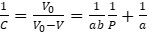(1)

where C is the rate of change in volume, V0 is the initial apparent volume (m3), V is the volume (m3) of powders under applied pressure, P is compacting pressure (MPa), and a and b are constants.

The Heckel equation is as follows,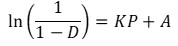(2)

where D is the relative density of the pellets (kg/m3), P is compacting pressure (MPa), and K and A are constants for a specific compacting system.

The Peiyun Huang equation is as follows,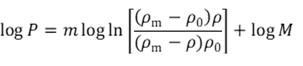(3)

where P is compacting pressure (MPa), ρ0 is the initial apparent density (kg/m3), ρm is the theoretical density (kg/m3), ρ is the pellet density (kg/m3), and m and M are constants for a specific compacting system.

Based on Eq. (1), Eq. (2), and Eq. (3), plots of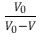vs. 1/P,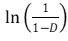vs. P, and log(P) vs.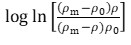are obtained and fitted to straight lines, respectively. Values of the constants are calculated from the slope and y-intercept of each fit.

This study applied the Kawakita equation, Heckel equation, and Peiyun Huang equation in the pelletizing process of rice straw, wheat straw, and wood shavings, under different levels of moisture content, compacting velocity, and particle size. The compacting forces were tested with a single pellet unit. The data fitted with the three equations were evaluated by regression analysis. Finally, effects of these factors on the coefficients in the three compression equations were discussed.

EXPERIMENTAL

Equipment and Materials

Device

Previously, a test device was developed to produce one pellet at a time (Wang et al. 2020a). As shown in Fig. 1, biomass particles were placed in the single pellet channel and compacted by the piston at a constant velocity until the pressure reached the maximum. The change of pressure during the compacting process was recorded. In this study, the dimension of the pellet channel was 20 mm; the weight of biomass in a single test was 8 g ± 0.1 g; and the maximum force applied on the piston was 20 kN.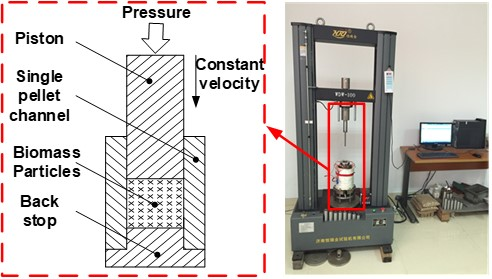Fig. 1. Test device with a single pellet unit

Test materials

The test materials were wheat straw, rice straw, and wood shavings. The wheat straw was collected in Yangzhou City, China, in June of 2020. The rice straw was collected in Nanjing City, China, in January of 2021. The wood shavings were wastes of house decoration obtained in Nanjing City, China, in June of 2019. The raw materials were kept in black sealed bags.

Based on the design of experiments, test materials were prepared. The raw materials were first crushed into particles, and then divided into fine and coarse groups by sieving. Two sieves with hole sizes of 2.5 mm and 1.25 mm were used. Materials passing through the 2.5 mm sieve and staying above the 1.25 mm sieve are collected as the coarse materials. Materials passing through the 1.25 mm sieve were collected as the fine materials. As a result, the ranges of the particle size for the fine and coarse materials were 0 mm to 1.25 mm and 1.25 mm to 2.5 mm, respectively. The crushed materials were separated into different groups with the weight of 8 g ± 0.1 g. The moisture content of each group was adjusted to the target with an oven and an electronic scale. Finally, these materials were sealed in separate black plastic bags and kept under room temperature for 24 h before their use.

Design of Experiments

Effects of moisture content, compacting velocity, and raw material particle size were considered in this study. Three groups of experiments were conducted. Only one factor changed in each group. For the first group, there were five different moisture contents (5% ± 1%, 10% ± 1%, 15% ± 1%, 20% ± 1%, and 25% ± 1%). The particle size was fine, and the compacting velocity was 80 mm/min. For the second group, there were three different compacting velocities (20 mm/min, 50 mm/min, and 80 mm/min). For the third group, there were two particle sizes (fine and coarse). The moisture content was 10% ± 1%, and the compacting velocity was 80 mm/min. The particle size was fine, and the moisture content was 10% ± 1%. Every trial was conducted three times.

For the approximation of ρm in Eq. (3), the three materials were compressed under the maximum compacting force of 20 kN and 80 kN (moisture content:10%, particle size: fine, compacting velocity: 80 mm/min), respectively. Based on the test data, an average ratio of the pellet density under 80 kN to that under 20 kN was obtained as 1.1771. The value of the ρm is the pellet density for each trial multiplied by 1.1771, respectively.

Statistical Analysis

The Kawakita equation, Heckel equation, and Peiyun Huang equation were used to fit the test data by the method of generalized least squares (Matlab, Mathworks, R2017-b, Natick, MA, USA). The coefficients of determination (R2) and regression coefficients for each fit were calculated, respectively.

RESULTS AND DISCUSSION

Compacting Force and Compression Equations

All the compacting force curves in this study had similar shapes. Normally, the densification process has been divided into compression and relaxion parts (Wang et al. 2020b; Caicedo-Zuñiga et al. 2021). However, details of the compression part have not been discussed. In this study, each curve was divided into four parts, as shown in Fig. 2. The compacting process began from part 1, in which no obvious increase in the compacting force was observed. The bulk density of the test materials was very low. In part 2, the compacting force began to increase, but the change rate was not fast. In part 3, the compacting force increased until it reached the maximum (20 kN). Part 4 was the relaxation period. The piston was held still, and the compacting force decreased. Therefore, the compression equations were discussed based on the data in part 2 and part 3.

As an example, one group of test data was analyzed with the three compression equations. The data were first processed by Kawakita equation, as shown in Fig. 3. Linear features are observed on both sides of the curve. However, there is a point of inflection when the compacting force is within the range 0.5 to 5 kN, which indicates the demarcation of parts 2 and 3. Because the X-axis of the curve is 1/P, the points are denser with the increase of compacting force. Therefore, in contrast to the conclusion drawn by Mani et al. (2004), the Kawakita equation is unfavorable for presenting details of the compacting process near the end. As a result, the Kawakita equation is not preferred for the mechanical behavior of biomass pelletizing process.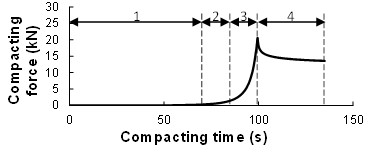Fig. 2. The curve of compacting force in a single trial

Then, the data were processed by the Heckel equation, as shown in Fig. 4. There was also a point of inflection when the compacting force was within the range 0.5 to 3 kN. Moreover, linear features are observed when the compacting force was over 3 kN.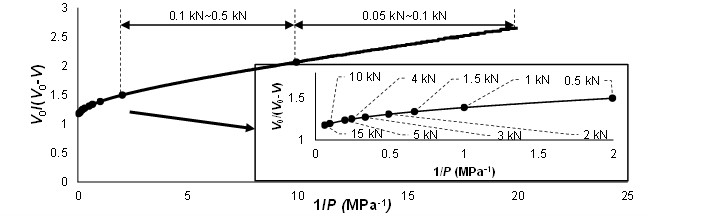Fig. 3. Curve of the data processed by Kawakita equation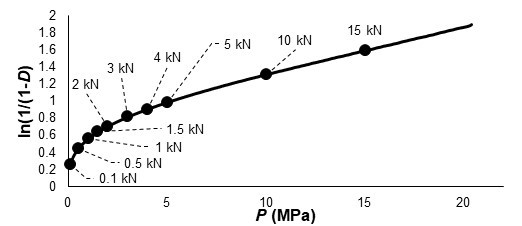Fig. 4. Curve of the data processed by Heckel equation

Finally, the data were processed by the Peiyun Huang equation, as shown in Fig. 5. Similar to Fig. 4, linear features were observed in the part under higher compacting force, and a point of inflection was observed when the compacting force was below 0.5 kN.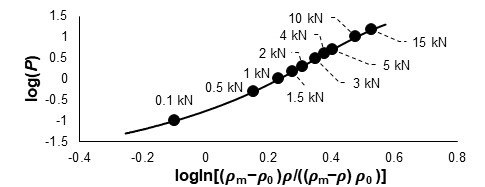Fig. 5. Curve of the data processed by Peiyun Huang double logarithm equation

According to the analysis above, all the three compression equations are suitable for part of the biomass pelletizing process. However, it seems inappropriate to fit the whole curve with a linear equation due to the inflection points in it. This is why Mani et al. (2004) drew the conclusion that the Heckel equation could not fit the densification process. The data were fitted with the three equations to discuss the possibility to model the compacting force with a specific equation. Since the compacting force near the end has greater effect on the pellet density, the ranges of the fitted data were narrowed gradually from the whole to the end. Coefficients of determination for the regressions are plotted in Fig. 6.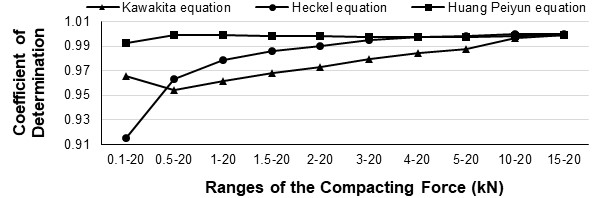Fig. 6. Coefficients of determination for the regressions of the data in different ranges

The values were over 0.95 when the ranges were within 0.5 kN to approximately 20 kN, which indicates the significance of the linear regressions. For the Kawakita equation and Heckel equation, the values of coefficients increased and approached 1 when the fitted data got close to the end. The coefficients were over 0.98 when the range was within 4 kN to approximately 20 kN. For the Peiyun Huang equation, all the values were over 0.99 and showed little change. Therefore, the Peiyun Huang equation was judged to be suitable for the whole compacting process, and Kawakita equation and Heckel equation were judged to be suitable for the compacting process under high compacting forces. According to the assumptions of the three equations, viscous properties are only considered in Peiyun Huang equation. Thus, the biomass materials used in this study should be a kind of visco-elasto-plastic powder (Caicedo-Zuñiga et al. 2021), but the effects of viscosity are reduced with the decrease of porosity.

Effects of Moisture Content on Compression Equations

The data were processed by the three compression equations separately. The coefficients of determination (R2) and regression coefficients of each equation for the materials under different moisture contents were obtained and are presented in Table 1. Results show that all the coefficients of determination were over 0.98, which indicates that the data were well fitted by the three equations. The effects of moisture content on the regression coefficients of the three equations are plotted in Fig. 7. Change rates of the coefficients with every 5% increase in the moisture content are included in Fig. 7.

Table 1. Fitting Results under Different Moisture Contents (Particle size: Fine, Compacting velocity: 80 mm/min)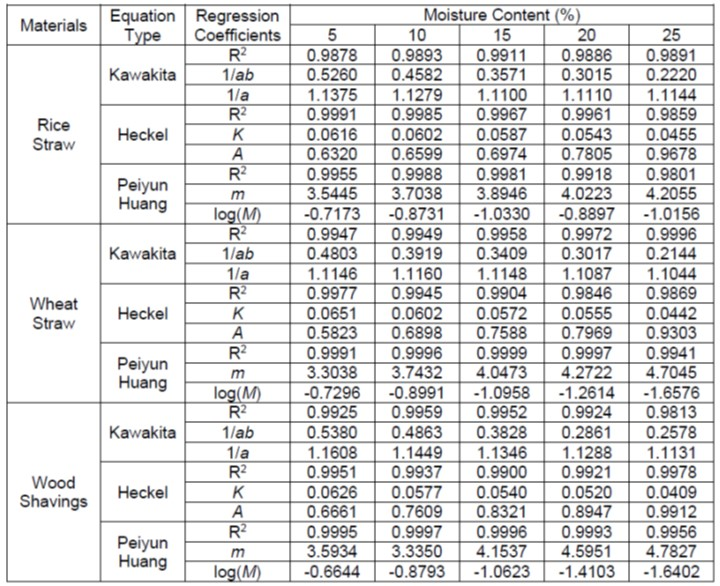For the Kawakita equation, moisture contents changed the 1/ab, but little effect on the 1/a, according to Fig. 7(a) and (b). The 1/ab decreased monotonically with the moisture content. This means that a higher density of the compressed biomass will be obtained at the same compression pressure when the moisture content increases. This is because water is a lubricant in the biomass compression process. The friction force between the biomass and die wall decreases with the moisture content (Guo et al. 2016). More effective force will be used to compress the biomass rather than overcome the friction force (Wang et al. 2020a).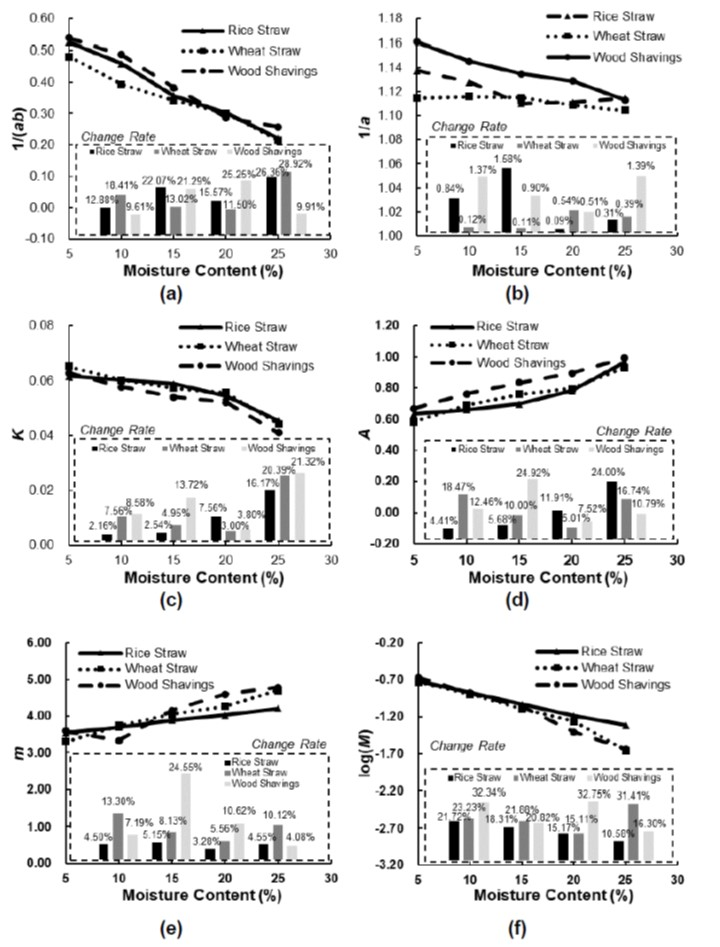Fig. 7. Effects of the moisture content on the coefficients of Kawakita equation, Heckel equation, and Peiyun Huang equation: (a) the coefficient of 1/(ab); (b) the coefficient of 1/a; (c) the coefficient of K; (d) the coefficient of A; (e) the coefficient of m; (f) the coefficient of log(M)

For the Heckel equation, the K decreased with the moisture content, as shown in Fig. 7(c). It showed a higher change rate of compression pressure with the increase of the moisture content, which is consistent with that of the Kawakita equation. The A increased with the moisture content as shown in Fig. 7(d). This means the bulk density of the biomass increased with the moisture content when the compression pressure equals zero. This is because the density of water is larger than that of biomass in this test.

For the Peiyun Huang equation, the m increased and the log(M) decreased with the moisture content, except at one point for wood shavings when the moisture content was 10%, as shown in Fig. 7(e) and (f). This phenomenon is similar to that of the Heckel equation, which means a higher change rate of the compression pressure.

Above all, the moisture content has monotonical effects on the coefficients. Lower pressure is required for the same pellet density when the moisture content increases. However, according to other studies, the effects of moisture contents on pellet qualities are not monotonical.

There will always be an optimal moisture content for a specific biomass to achieve high pellet density and strength, which means too low or too high moisture content are not suitable for biomass pelletizing processes (Guo et al. 2016; Chen et al. 2021; Cui et al. 2021; Lavergne et al. 2021). Furthermore, values of the fitted coefficients for different materials are close to each other under some moisture contents. It is possible that different biomass materials under specific moisture contents may share the same compacting equation. Therefore, it is unworkable to distinguish the types of biomass by the coefficient in compression equations. Furthermore, the pellet quality cannot be predicted just based on the coefficients.

Effects of Compacting Velocity on Compression Equations

The data were processed by the three compression equations separately. The coefficients of determination (R2) and regression coefficient of each equation for the materials under different compacting velocities were obtained and presented in Table 2. All the coefficients of determination were over 0.98, and most of the values were over 0.99. Therefore, the three equations fit the data well.

The effects of compacting velocity on the regression coefficients of the three equations are plotted in Fig. 8. Change rates of the adjacent points are calculated and presented in Fig. 8. For the Kawakita equation, the increasing velocity did not change the 1/a according to Fig. 8(b), because most of the maximum change rates were under 1%. This was similar to the effects of moisture contents. However, the effects of compacting velocity on the 1/ab were different for the three materials. The values decreased for the rice straw, increased for the wood shavings, but changed little for the wheat straw, as shown in Fig. 8(a).

For the Heckel equation and Peiyun Huang equation, all the coefficients changed differently when the compacting velocity increased. For example, the log(M) increased for the wood shavings, decreased for the rice straw, but changed little for the wheat straw, as presented in Fig. 8(f). Therefore, no common rules could be drawn for the effects of the compacting velocity on these coefficients.

Table 2. Fitting Results under Different Compacting Velocities (Particle size: Fine, Moisture: 10%)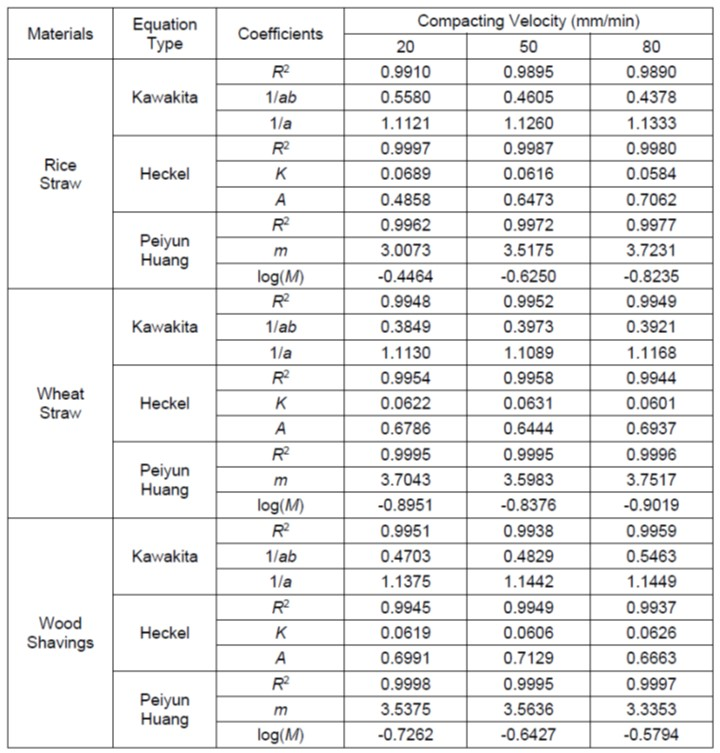However, if Fig. 8(c) was compared with Fig. 8(e), it could be found that the K and m changed in opposite trends. A similar phenomenon was observed for A and log(M), as shown in Fig. 8(d) and (f). Thus, the Heckel equation and Peiyun Huang equation are similar in form when dealing with biomass densification process.

Based on an overall view of Fig. 8, the compacting velocity had less effects on the coefficients compared with the moisture content. This means that the compacting velocity had less influence than the moisture. The velocity primarily affects the productivity rather than the pellet quality (Xia et al. 2014). Moreover, the rice straw was more sensitive to the velocity compared with the wheat straw and wood shavings.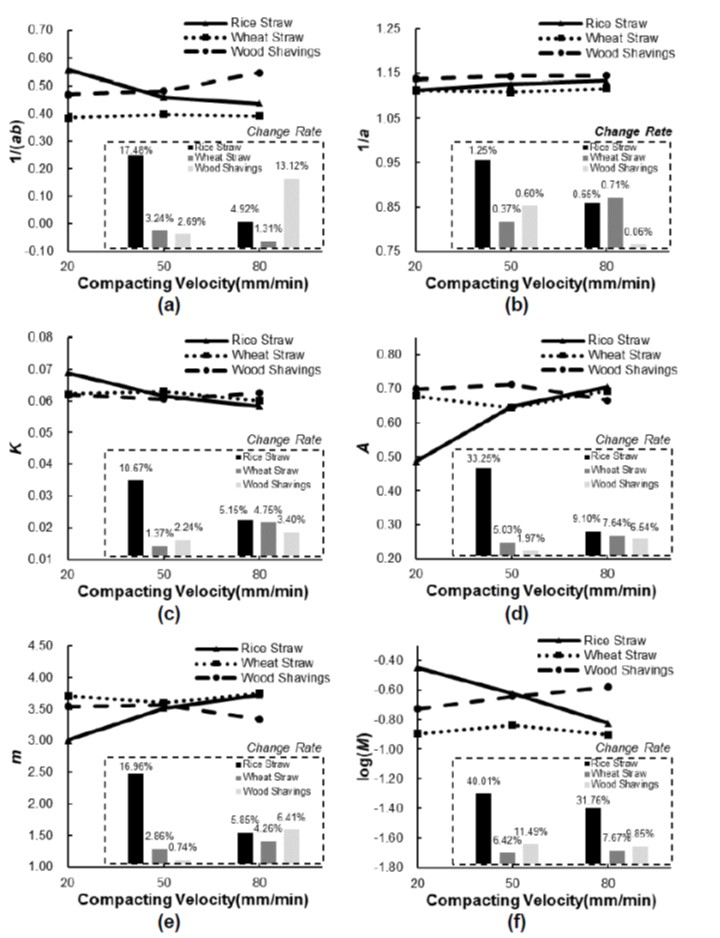Fig. 8. Effects of the compacting velocity on the coefficients of Kawakita equation, Heckel equation, and Peiyun Huang equation: (a) the coefficient of 1/(ab); (b) the coefficient of 1/a; (c) the coefficient of K; (d) the coefficient of A; (e) the coefficient of m; (f) the coefficient of log(M)

Effects of Raw Material Particle Size on Compression Equations

The data were processed by the three compression equations separately. The coefficients of determination (R2) and regression coefficient of each equation for the materials under different particle sizes were obtained and are presented in Table 3. The three equations fit the data well because almost all the coefficients of determination were over 0.99. The effects of particle size on the regression coefficients of the three equations are plotted in Fig. 9. Change rates of the test data are illustrated in Fig. 9.

Table 3. Fitting Results under Different Raw Material Particle Sizes (Moisture: 10%, Compacting velocity: 80 mm/min)